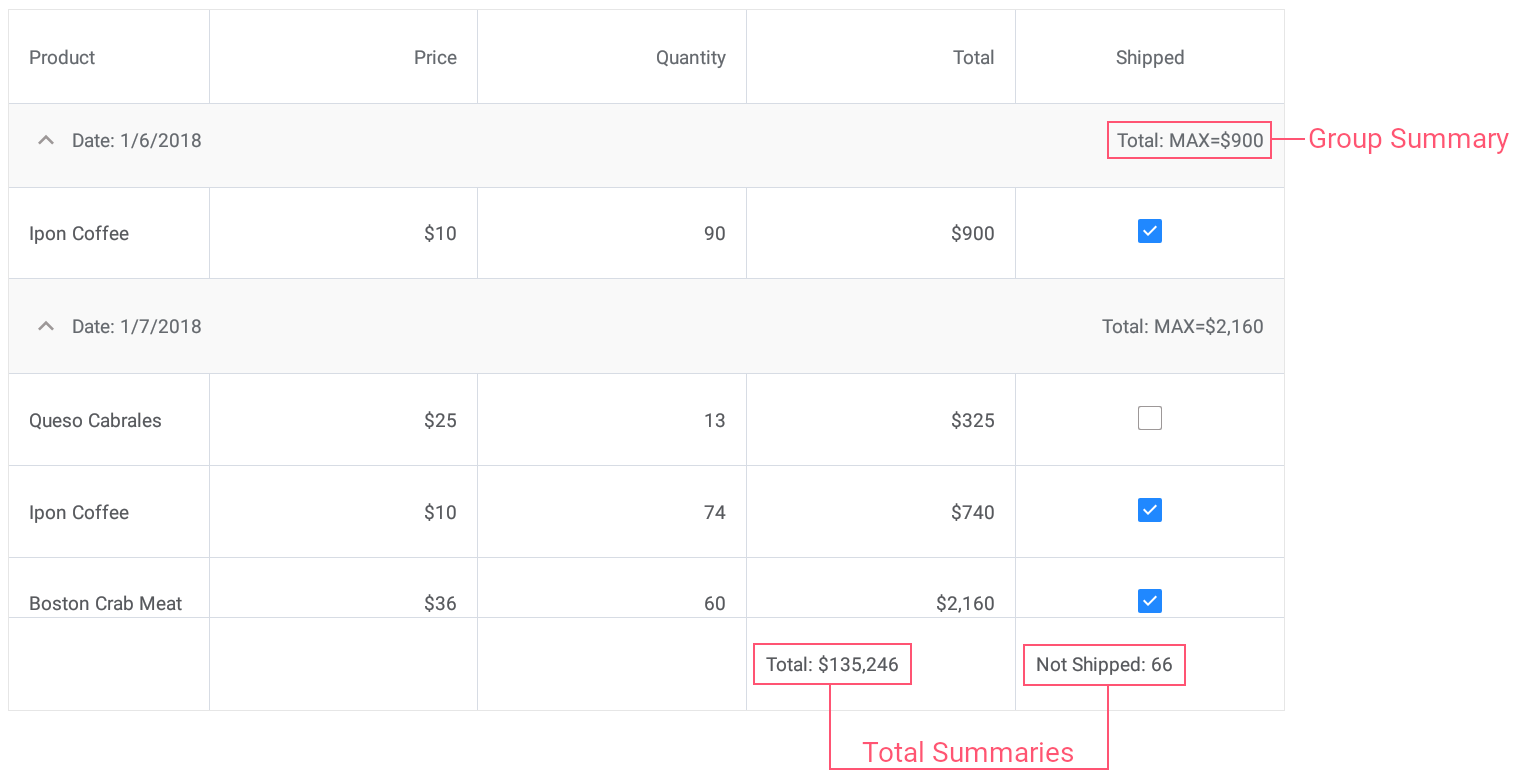21.1
20.2
20.1
19.2
19.1
1.1
The page you are viewing does not exist in version 1.1. This link will take you to the root page.

# CustomSummaryEventArgs.SummaryProcess Property

Gets a value that indicates the data summary calculation stage.

Namespace: DevExpress.XamarinForms.DataGrid

Assembly: DevExpress.XamarinForms.Grid.dll

## Declaration

``public CustomSummaryProcess SummaryProcess { get; }``

## Property Value

Type Description
CustomSummaryProcess

A value indicating the calculation stage.

Available values:

Name Description
Start

Indicates that the process of custom summary calculation is about to start.

Calculate

Indicates that custom summary calculation is in progress. This means the event is raised for a specific data row that contains the field value used to calculate the processed custom summary.

Finalize

Indicates that the process of custom summary calculation is finished.

## Remarks

The process of custom summary calculation consists of three stages.

## Example

This example demonstrates how to use predefined aggregate functions and custom rule to calculate group and total summaries for grid columns.• Set the group summary to display the maximum Total value for each group of records.
• Set the total summary to calculate the sum of values in the Total column.
• Set the custom total summary to count the number of orders whose value in the Shipped column is false (to count orders that are not shipped).
``````<dxg:DataGridView x:Name="grid" ItemsSource="{Binding Orders}"
CalculateCustomSummary="OnCalculateCustomSummary">
<!-- ... -->
<dxg:DataGridView.GroupSummaries>
<dxg:GridColumnSummary FieldName="Total" Type="Max"/>
</dxg:DataGridView.GroupSummaries>

<dxg:DataGridView.TotalSummaries>
<dxg:GridColumnSummary FieldName="Total" Type="Sum"
DisplayFormat="Total: {0:C0}"/>
<dxg:GridColumnSummary FieldName="Shipped" Type="Custom"
DisplayFormat="Not Shipped: {0}"/>
</dxg:DataGridView.TotalSummaries>
</dxg:DataGridView>
``````
``````int count;
// ...

void OnCalculateCustomSummary(object sender, CustomSummaryEventArgs e) {
if (e.FieldName.ToString () == "Shipped")
if (e.IsTotalSummary){
if (e.SummaryProcess == CustomSummaryProcess.Start) {
count = 0;
}
if (e.SummaryProcess == CustomSummaryProcess.Calculate) {
if (!(bool)e.FieldValue)
count++;
e.TotalValue = count;
}
}
}
``````
See Also